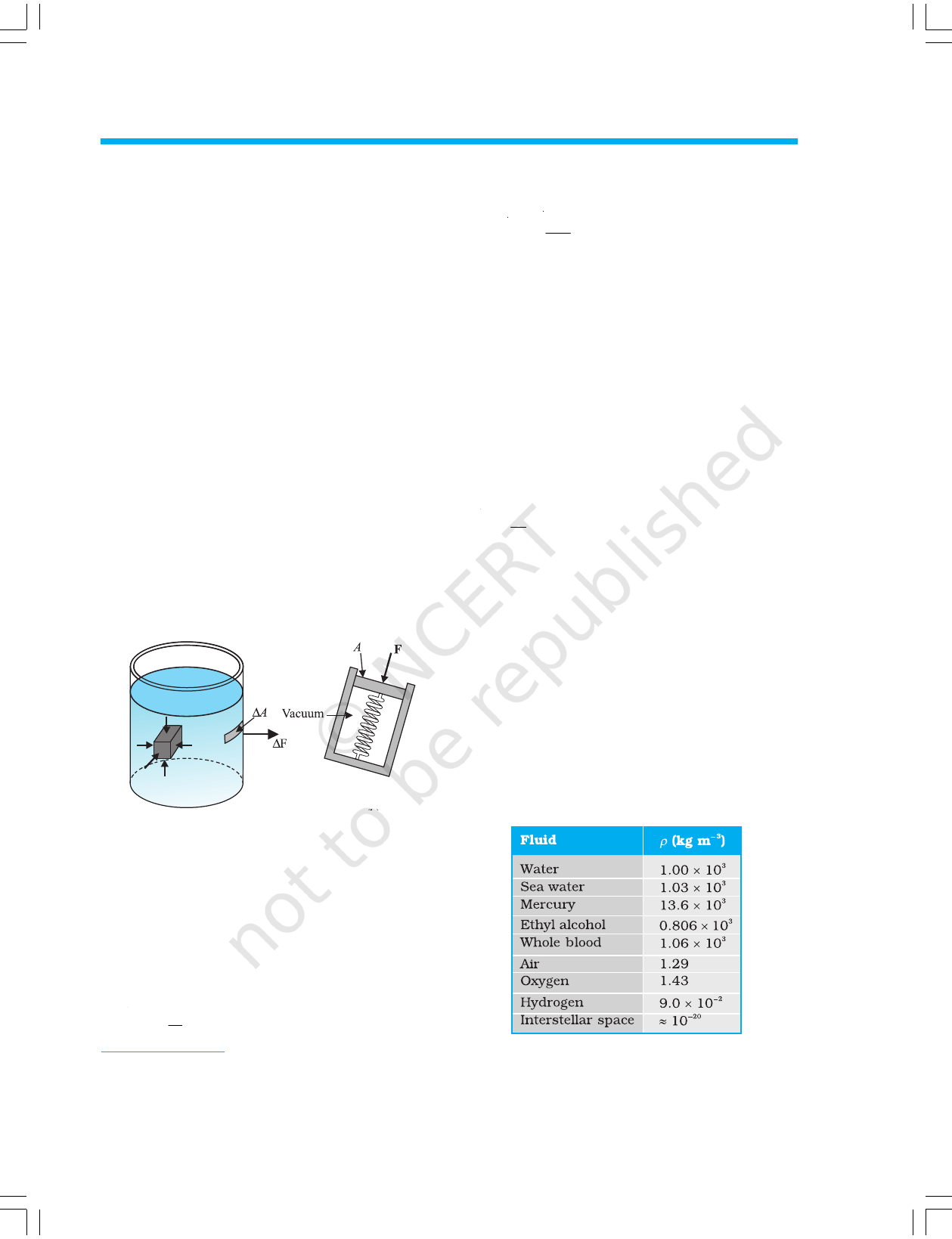CHAPTER TEN
MECHANICAL PROPERTIES OF FLUIDS
10.1 INTRODUCTION
In this chapter, we shall study some common physical
properties of liquids and gases. Liquids and gases can flow
and are therefore, called fluids. It is this property that
distinguishes liquids and gases from solids in a basic way.
Fluids are everywhere around us. Earth has an envelop of
air and two-thirds of its surface is covered with water. Water
is not only necessary for our existence; every mammalian
body constitute mostly of water. All the processes occurring
in living beings including plants are mediated by fluids. Thus
understanding the behaviour and properties of fluids is
important.
How are fluids different from solids? What is common in
liquids and gases? Unlike a solid, a fluid has no definite
shape of its own. Solids and liquids have a fixed volume,
whereas a gas fills the entire volume of its container. We
have learnt in the previous chapter that the volume of solids
can be changed by stress. The volume of solid, liquid or gas
depends on the stress or pressure acting on it. When we
talk about fixed volume of solid or liquid, we mean its volume
under atmospheric pressure. The difference between gases
and solids or liquids is that for solids or liquids the change
in volume due to change of external pressure is rather small.
In other words solids and liquids have much lower
compressibility as compared to gases.
Shear stress can change the shape of a solid keeping its
volume fixed. The key property of fluids is that they offer
very little resistance to shear stress; their shape changes by
application of very small shear stress. The shearing stress
of fluids is about million times smaller than that of solids.
10.2 PRESSURE
A sharp needle when pressed against our skin pierces it. Our
skin, however, remains intact when a blunt object with a
wider contact area (say the back of a spoon) is pressed against
it with the same force. If an elephant were to step on a man’s
chest, his ribs would crack. A circus performer across whose
10.1 Introduction
10.2 Pressure
10.3 Streamline flow
10.4 Bernoulli’s principle
10.5 Viscosity
10.6 Surface tension
Summary
Points to ponder
Exercises
Appendix
2020-21chest a large, light but strong wooden plank is
placed first, is saved from this accident. Such
everyday experiences convince us that both the
force and its coverage area are important. Smaller
the area on which the force acts, greater is the
impact. This impact is known as pressure.
When an object is submerged in a fluid at
rest, the fluid exerts a force on its surface. This
force is always normal to the object’s surface.
This is so because if there were a component of
for
ce parallel to the surface, the object will also
exert a force on the fluid parallel to it; as a
consequence of Newton’s third law. This force
will cause the fluid to flow parallel to the surface.
Since the fluid is at rest, this cannot happen.
Hence, the force exerted by the fluid at rest has
to be perpendicular to the surface in contact
with it. This is shown in Fig.10.1(a).
The normal force exerted by the fluid at a point
may be measured. An idealised form of one such
pressure-measuring device is shown in Fig.
10.1(b). It consists of an evacuated chamber with
a spring that is calibrated to measure the force
acting on the piston. This device is placed at a
point inside the fluid. The inward force exerted
by the fluid on the piston is balanced by the
outward spring force and is thereby measured.
If F is the magnitude of this normal force on the
piston of area A then the average pressure P
av
is defined as the normal force acting per unit
area.
P
F
A
av
=
(10.1)
In principle, the piston area can be made
arbitrarily small. The pressure is then defined
in a limiting sense as
P =
lim
A 0
F
A
(10.2)
Pressure is a scalar quantity. We remind the
reader that it is the component of the force
normal to the area under consideration and not
the (vector) force that appears in the numerator
in Eqs. (10.1) and (10.2). Its dimensions are
[ML
–1
T
–2
]. The SI unit of pressure is N m
–2
. It has
been named as pascal (Pa) in honour of the
French scientist Blaise Pascal (1623-1662) who
carried out pioneering studies on fluid pressure.
A common unit of pressure is the atmosphere
(atm), i.e. the pressure exerted by the
atmosphere at sea level (1 atm = 1.013 × 10
5
Pa).
Another quantity, that is indispensable in
describing fluids, is the density
ρ
. For a fluid of
mass m occupying volume V,
ρ
=
m
V
(10.3)
The dimensions of density are [ML
–3
]. Its SI
unit is kg m
–3
. It is a positive scalar quantity. A
liquid is largely incompressible and its density
is therefore, nearly constant at all pressures.
Gases, on the other hand exhibit a large
variation in densities with pressure.
The density of water at 4
o
C (277 K) is
1.0 × 10
3
kg m
–3
. The relative density of a
substance is the ratio of its density to the
density of water at 4
o
C. It is a dimensionless
positive scalar quantity. For example the relative
density of aluminium is 2.7. Its density is
2.7 × 10
3
kg m
–3
.
The densities of some common
fluids are displayed in Table 10.1.
Table 10.1 Densities of some common fluids
at STP*
(a) (b)
Fig. 10.1 (a) The force exerted by the liquid in the
beaker on the submerged object or on the
walls is normal (perpendicular) to the
surface at all points.
(b) An idealised device for measuring
pressure.
* STP means standard temperature (0
0
C) and 1 atm pressure.
MECHANICAL PROPERTIES OF FLUIDS 251
2020-21